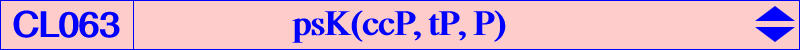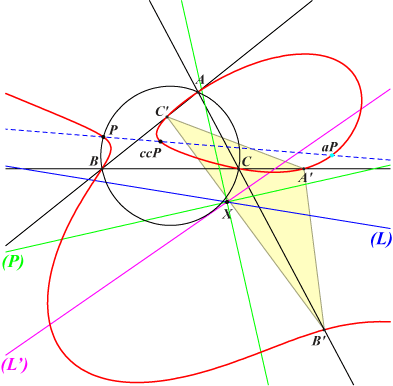Let P = p : q : r be a point. A member K(P) of CL063 is a cubic psK(ccP, tP, P) as in Pseudo-Pivotal Cubics and Poristic Triangles, where ccP is the complement of the complement of P and tP the isotomic conjugate of P. K(P) is always a K+ i.e. a cubic with concurring asymptotes at a point X = (q + r)(2p + q + r)[p(p + q + r) + 3 q r] : : . When P lies on the line at infinity, it becomes a K++ since X lies on the curve. In this case, the cubic is a trident. See below for further details. Each cubic K(P) of CL063 is actually the barycentric quotient of a member of CL017 by P. For example, K208 in CL017 corresponds to K071 in CL063 : the barycentric quotient of each point on K208 by P = X(4) is a point on K071. Similarly, K207 corresponds to K702 = psK(X1125, X75, X1) with P = X(1). In this latter case, the trilinear equation of K207 is the barycentric equation of K702. *** Geometric properties of K(P) K(P) is a circum-cubic passing through P, aP (anticomplement of P), ccP, tctP, the vertices of the cevian triangle of tP. Its equation is : ∑ q r x (y + z) [q y - r z + 2(r y - q z)] + p q r (y - z)(z - x)(x - y) = 0. It meets the line at infinity at the same points as the three cubics Ki below and meets each cubic again at 3 finite points (apart A, B, C) lying on the circum-conic Ci with perspector Pi. K1 = pK(P x ccP, P), P1 = p(q - r)(2p + q + r) : : , K2 = pK(ctP, cP), P2 = p^2(q - r) : : , K3 = pK(cP x ccP, aP), P3 = (q - r)(- p + q + r)(2p + q + r) : : . K(P) meets the Steiner ellipse at the same points as the cubic K4 = pK(tP, ccP) and the three other common points of the two cubics lie on the line : ∑ p(q - r)(p +2 q + 2 r) x = 0.K(P) with P on the line at infinity K(P) is a trident and then X = P which is a node on the cubic. K(P) has two asymptotes : one is the line (A) passing through G and P, the other is a parabola (P) passing through G with axis parallel to GP. The figure shows K(X514), a trident passing through X(514), X(1016), X(1019). See CL029 and CL070 for other tridents which are cKs and psKs respectively.K(P) with P on the circumcircle K(P) is a cubic having a pencil of circular polar conics. The polar conic of each point on the line (L') is a circle and all these circles are in a same pencil. (L') is called the circular line of the cubic. See other similar cubics in Table 47. In such case, the poloconic of the line at infinity splits into two perpendicular lines secant at X and (L') is the reflection of the orthic line (L) about any of these lines. Obviously, the polar conic of X is not a proper circle but the union of the line at infinity and the radical axis of the pencil above.Special cubics K(P) K(P) is equilateral if and only if P = X(4). The corresponding cubic is K071. K(P) is circular if and only if P is one of the points Ua, Ub, Uc described in the page K001. These points are the images under h(X4, 2) of the intersections (other than A, B, C) of the circumcircle and the Napoleon cubic K005.Examples of cubics K(P) The following table gives a selection of these cubics.P K(P) / centers on K(P) remarks X(1) K702 / X(1), X(8), X(79), X(274), X(1125) X(3) psK(X140, X264, X3) / X(3), X(4), X(140), X(276) X(4) K071 / X(4), X(5), X(20), X(76) equilateral X(7) psK(X142, X8, X7) / X(7), X(75), X(142), X(144), X(3059) X(8) psK(X10, X7, X8) / X(8), X(10), X(65), X(85), X(145) X(20) psK(X3, X253, X20) / X(3), X(20), X(64), X(3146) X(74) psK(cX113, X3260, X74) / X(74), X(146), X(265) note 1 X(99) psK(X620, X523, X99) / X(99), X(148), X(620) note 1 X(100) psK(X3035, X693, X100) / X(100), X(149), X(3035) note 1 X(514) psK(X514, X190 , X514) / X(514), X(1016), X(1019) tridentnote 1 : K(P) is a cubic having a pencil of circular polar conics.